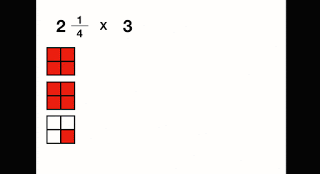## Thursday, 21 March 2019

### Keynote Fraction Animations

A really boring title I know, but this activity was lots of fun and was a great example of learning and understanding concepts rather than just knowing answers.

We are studying fractions in grade 6, in my experience students often know shortcuts and tricks to do equations with fractions

While this approach gives them an answer it doesn't promote understanding, rather it focuses on answers. I am much more interested in students understanding a concept rather than knowing an answer. Often they don't understand what the equations actually mean and written explanations are complicated and confusing.

There are lots of great visual examples online and sites like Myimaths have animations and videos explaining what is actually happening when you add, subtract, divide or multiply a fraction. While these sites promote understanding and make it easy for students to see what it means to solve equation problems, I prefer making my own or even better get the students creating their own fraction animations.Keynote Fraction Animation

To achieve this we used Keynote and added actions to a variety of shapes and text boxes so that students could create their own fraction animations. About half way through the activity I remembered magic move and that made things so much easier.

Magic move compares one slide to the next, takes note of any changes and then animates the changes. You can easily duplicate a slide, make some changes and turn it into a nifty animation in no time at all.

Here are a series of videos I created on how to make your own fraction animations the first two use regular animations and the second two (multiplication and division use magic move)

Subtracting Fractions
Multiplying Fractions

Dividing Fractions

What I really like about this activity is that it is hard work, the students really need to think about what it means to perform operations on fractions. What does it mean to add fractions beyond a set of rules.

They look at fractions and operations in different ways, work backwards and need to problem solve to make their animations reflect what is actually happening when performing operations on fractions.

#### 1 comment:

1.Multiplying and dividing fractions is time consuming and can exhaust too much energy on thinking what is the right answer. If practicing how to multiply or add fractions, it is better to do it manually but when you are in an actual situation that you are doing arithmetic operation, it is better to use a fraction calculator. Using fraction calculator not bad and it is a good strategy to save your time and physical strength plus you can be sure that you got the correct answer.